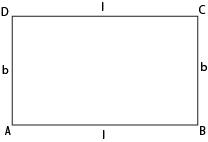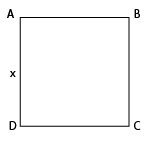Volume of a cuboid

## Objective

To obtain a formula for finding the volume of a cuboid.

## What is Cube and Cuboid?

Cube and cuboid shapes in Math are three-dimensional shapes. The cube and cuboid are obtained from the rotation of the two-dimensional shapes called square and rectangle respectively.

Cube: A cube is a three-dimensional shape. It has six faces, eight vertices and twelve edges. All the faces of the cube are in square shape and have equal dimensions.

Cuboid: A cuboid is also a polyhedron having six faces, eight vertices and twelve edges. The faces of the cuboid are parallel. But not all the faces of a cuboid are equal in dimensions.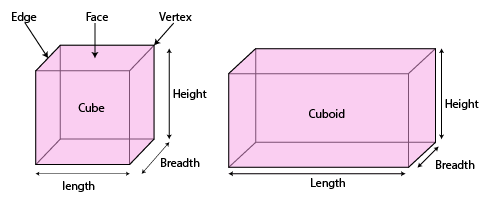## What is Volume?

• If an object is solid, then the space occupied by such an object is measured and is termed the volume of the object.
• On the other hand, if the object is hollow, then the interior is empty and can be filled with air, or some liquid that will take the shape of its container. In this case, the volume of the substance that can fill the interior is called the capacity of the container.
• In short, the volume of an object is the measure of the space it occupies, and the capacity of an object is the volume of substance its interior can accommodate.
• Hence, the unit of measurement for either of the two is a cubic unit.
• The volume of the cuboid depends on its length, breadth, and height, hence changing any one of those quantities changes the volume of the shape.

## Volume of Cuboid

The formula for the volume of the cuboid can be derived from the concept explained on rectangular sheets.

Let the area of a rectangular sheet of paper be 'A', the height up to which they are stacked be 'h' and the volume of the cuboid be 'V'.

Can you tell what would be the relationship between V, A, and h?

The area of the plane region occupied by each rectangle × height = Measure of the space occupied by the cuboid.

So, we get A × h = V

That is,

The volume of cuboid = Base area × Height

The base area for cuboid = l × b

Hence, the volume of a cuboid, V = l × b × h = lbh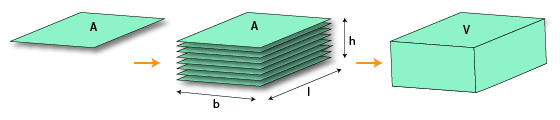for example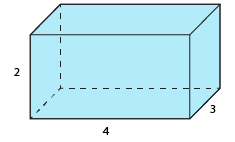Let a cuboid of length = 4 units , breadth = 3 units and height = 2 units
Then, by the formula, Volume of the cuboid = (length × breadth × height) cubic units.
So, we get the volume as = 4 x 3 x 2 = 24 cubic units.

## Prerequisite knowledge

Students should already be familiar with

• Rules of multiplication
• Area of rectangle and square

Concept of Area of rectangle:

Let us say a rectangle ABCD of
length=l